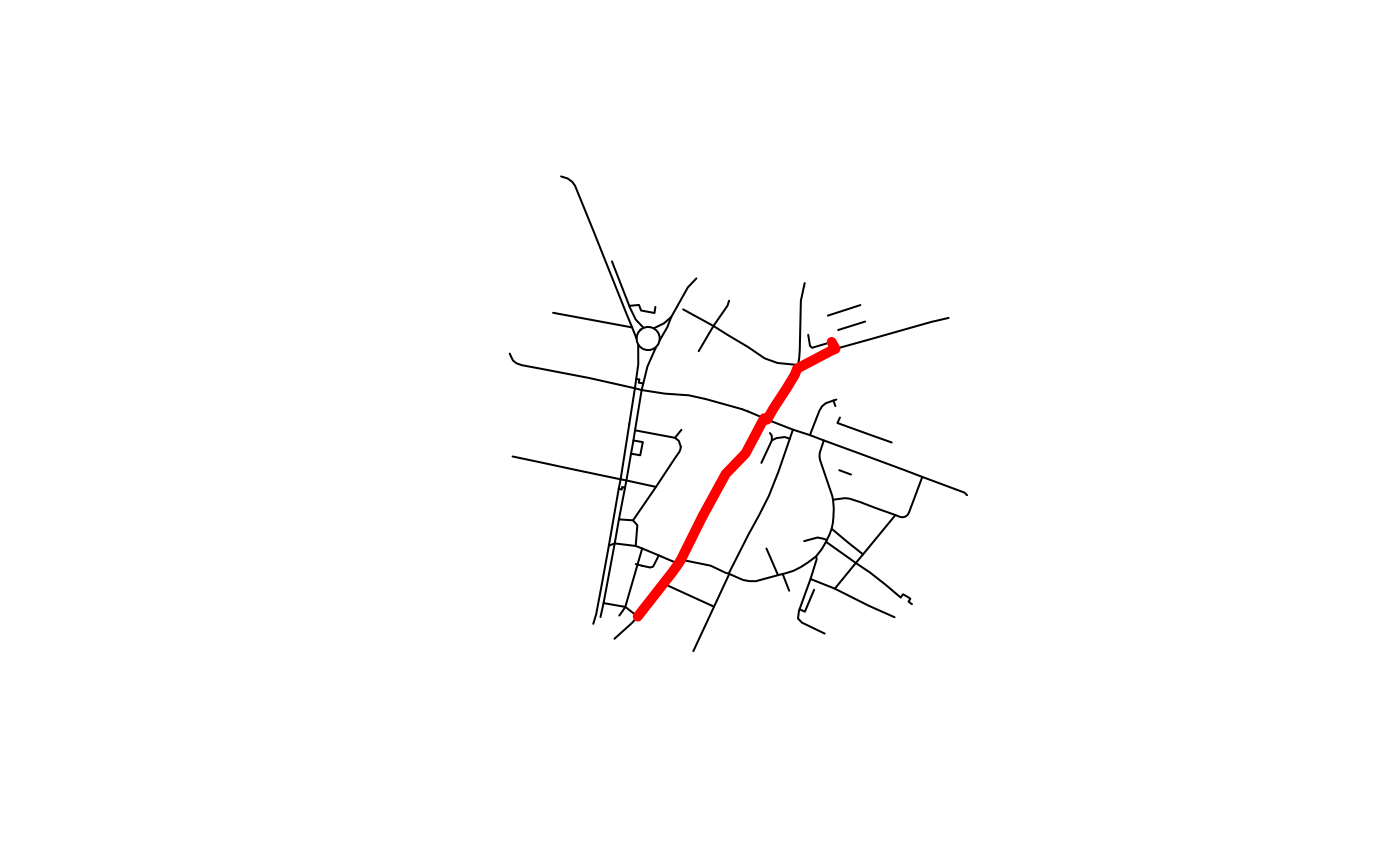Route on local data using the dodgr package

## Usage

``route_dodgr(from = NULL, to = NULL, l = NULL, net = NULL)``

## Arguments

from

An object representing origins (if lines are provided as the first argument, from is assigned to `l`)

to

An object representing destinations

l

A spatial (linestring) object

net

sf object representing the route network

Other routes: `route_osrm()`, `route()`

## Examples

``````if (requireNamespace("dodgr")) {
from <- c(-1.5327, 53.8006) # from <- geo_code("pedallers arms leeds")
to <- c(-1.5279, 53.8044) # to <- geo_code("gzing")
# next 4 lines were used to generate `stplanr::osm_net_example`
# pts <- rbind(from, to)
# colnames(pts) <- c("X", "Y")
# net <- dodgr::dodgr_streetnet(pts = pts, expand = 0.1)
# osm_net_example <- net[c("highway", "name", "lanes", "maxspeed")]
r <- route_dodgr(from, to, net = osm_net_example)
plot(osm_net_example\$geometry)
plot(r\$geometry, add = TRUE, col = "red", lwd = 5)
}